# 21. Connection¶

This notebook is part of the Introduction to manifolds in SageMath by Andrzej Chrzeszczyk (Jan Kochanowski University of Kielce, Poland).

In :
version()

Out:
'SageMath version 9.6, Release Date: 2022-05-15'

Roughly speaking, connection $∇$ on a smooth manifold $M$, is a rule to calculate the directional derivatives of the vector fields on $M$. If $X$ and $Y$ are two vector fields, $∇_X Y$ denotes the vector field whose value at each point $p ∈ M$ is equal to the directional derivative of $Y$ in the direction of $X_p$ .

### Euclidean connection in $U\subset R^n$¶

Let $U\subset R^n$ be an open subset and $\ \ (x^1,\ldots,x^n)\ \$ define Cartesian coordinates on $U$. For $X,Y\in \mathfrak{X}(U),$ $\ X=X^i\frac{\partial}{\partial x^i},\ Y=Y^i\frac{\partial}{\partial x^i}\$ define $\ D_XY\in \mathfrak{X}(U)\$ by

$$D_XY=X(Y^j)\frac{\partial}{\partial x^j}=X^i\frac{\partial Y^j}{\partial x^i}\frac{\partial}{\partial x^j}.$$

Thus, each component of the vector field $D_XY$ is the usual directional derivative in $R^n$ of $Y^j$ in the direction of $X$.

The map $$(X,Y)\to D_XY$$

is $R$-linear with respect to $Y$ since

$$X(\alpha Y_1^j+\beta Y_2^j)=\alpha X(Y_1^j)+\beta X(Y_2^j),\quad \text{for } \alpha,\beta\in R.$$

It is $C^\infty(U)$ -linear in $X$ since

$$(f_1X_1+f_2X_2)(Y^j)= f_1X_1(Y^j)+f_2X_2(Y^j), \ \text{for } \ f_1,f_2\in C^\infty(U).$$

It satisfies the Leibniz rule since

$D_XY(fY)=X(fY^j)\frac{\partial}{\partial x^j}= (Xf)Y^j\frac{\partial}{\partial x^j}+fX(Y^j)\frac{\partial}{\partial x^j} =(Xf)Y+fD_XY.$

### Affine connection in a manifold¶

The above observations motivate the following definition.

Let $M$ be a smooth manifold. A connection on $M$ assigns to each $X ∈ \mathfrak{X}(M)$ an operator $∇_X$ from $\mathfrak{X}(M)$ into itself, such that for all $X, Y, Z ∈ \mathfrak{X}(M), a, b ∈ R$ and $f ∈ C^∞ (M)$,

\begin{eqnarray} \tag{21.1} &∇_X (aY + bZ) = a∇_X Y + b∇_X Z,\\ \tag{21.2} &∇_X ( f Y) = f ∇_X Y + (X f )Y,\\ \tag{21.3} &∇_{aX+bY} Z = a∇_X Z + b∇_Y Z,\\ \tag{21.4} &∇_{f X} Y = f ∇_X Y. \end{eqnarray}

$∇_X Y$ is called the covariant derivative of $Y$ with respect to $X$.

If $\ (x^1 , x^2 , . . . , x^n )\$ is a coordinate system in some neighborhood $U$ of $M$, and $\ X = X^i \frac{∂}{∂ x^i},\ \ Y = Y^j \frac{∂}{∂ x^j},\$ then from (21.3),(21.4) it follows

$$\displaystyle \nabla_{X^i\frac{∂}{∂ x^i}}Y=\displaystyle \nabla_{(X^1\frac{∂}{∂ x^1}+\ldots+X^n\frac{∂}{∂ x^n})}Y \stackrel{\text{(3)}}{=}\displaystyle \nabla_{X^1\frac{∂}{∂ x^1}}Y+\ldots+\nabla_{X^n\frac{∂}{∂ x^n}}Y\\ \stackrel{\text{(4)}}{=}\displaystyle X^1\nabla_{\frac{∂}{∂ x^1}}Y+\ldots+X^n\nabla_{\frac{∂}{∂ x^n}}Y =X^i\nabla_{\frac{∂}{∂ x^i}}Y.$$

From (21.1),(21.2) we have $$\nabla_{\frac{∂}{∂ x^i}}Y= \nabla_{\frac{∂}{∂ x^i}}\big(Y^j\frac{∂}{∂ x^j}\big) =\nabla_{\frac{∂}{∂ x^i}}\big(Y^1\frac{∂}{∂ x^1}+\ldots Y^n\frac{∂}{∂ x^n}\big)\\ \stackrel{\text{(1)}}{=}\displaystyle \nabla_{\frac{∂}{∂ x^i}}\big(Y^1\frac{∂}{∂ x^1}\big)+\ldots+ \nabla_{\frac{∂}{∂ x^i}}\big(Y^n\frac{∂}{∂ x^n}\big)$$ $$\stackrel{\text{(2)}}{=} Y^1\nabla_{\frac{∂}{∂ x^i}}\big(\frac{∂}{∂ x^1}\big)+ \big(\frac{∂}{∂ x^i}Y^1\big)\frac{∂}{∂ x^1}+ \ldots+ Y^n\nabla_{\frac{∂}{∂ x^i}}\big(\frac{∂}{∂ x^n}\big)+ \big(\frac{∂}{∂ x^i}Y^n\big)\frac{∂}{∂ x^n}\\ =Y^j\nabla_{\frac{∂}{∂ x^i}}\frac{∂}{∂ x^j}+ \big(\frac{∂}{∂ x^i}Y^j\big)\frac{∂}{∂ x^j} =\big(\frac{∂}{∂ x^i}Y^j\big)\frac{∂}{∂ x^j} +Y^j\nabla_{\frac{∂}{∂ x^i}}\frac{∂}{∂ x^j}.$$

Thus (21.1)-(21.4) give us

$$∇_X Y=X^i\big[\big(\frac{∂}{∂ x^i}Y^j\big)\frac{∂}{∂ x^j} +Y^j\nabla_{\frac{∂}{∂ x^i}}\frac{∂}{∂ x^j}\big].$$

Since $\nabla_{\frac{∂}{∂ x^i}}\frac{∂}{∂ x^j}$ is a smooth vector field, it is a linear combination of the vector fields $\{\frac{∂}{∂ x^k}\}_{k=1}^n$, so there is a set of $n^3$ smooth functions $\displaystyle\Gamma^k_{ji}$ on $U$, such that

\begin{equation} \nabla_{\frac{∂}{∂ x^i}}\frac{∂}{∂ x^j}=\Gamma^k_{ji}\frac{∂}{∂ x^k}. \tag{21.5} \end{equation}

Functions $\displaystyle\Gamma^k_{ji}$ which are called Christoffel symbols define the connection in the coordinate system $(x^1,\ldots,x^n)$ since

\begin{equation} \begin{matrix} \displaystyle ∇_X Y=X^i\big[\big(\frac{∂}{∂ x^i}Y^j\big)\frac{∂}{∂ x^j} +Y^j\Gamma^k_{ji}\frac{∂}{∂ x^k}\big]\\ \displaystyle =X^i\big(\frac{∂}{∂ x^i}Y^k+ \Gamma^k_{ji}Y^j\big)\frac{∂}{∂ x^k}. \end{matrix} \tag{21.6} \end{equation}

Comparing (21.6) with the definition of the Euclidean connection we see that all Christoffel symbols for the Euclidean connection vanish.

Observe that if in the definition of the Euclidean connection, we put $X=\frac{∂}{∂ x^i}$ and $Y=\frac{∂}{∂ x^j}$, then the components of $Y$ in Cartesian coordinates are $(0,\ldots,1,\ldots,0),\$ ($1$ on $j$-th position) and $\displaystyle D_{\frac{∂}{∂ x^i}}\frac{∂}{∂ x^j}= \frac{∂}{∂ x^i}(0)\frac{∂}{∂ x^1}+\ldots+ \frac{∂}{∂ x^i}(1)\frac{∂}{∂ x^j}+\ldots+ \frac{∂}{∂ x^i}(0)\frac{∂}{∂ x^n}=0.$

Example 21.1

Consider the two-dimensional half-plane $y>0$ with connection coefficients defined by $\ \ \Gamma^1_{12}=\Gamma^1_{21}=\Gamma^2_{22}=-\frac{1}{y}\ \$ and $\ \ \Gamma^2_{11}=\frac{1}{y}.$

In :
%display latex
M = Manifold(2, 'M', start_index=1)        # manifold M, y>0
c_xy.<x,y> = M.chart()                     # chart on M
# affine connection on M
nab = M.affine_connection('nabla', r'\nabla') ; print(nab)

Affine connection nabla on the 2-dimensional differentiable manifold M

In :
                                           # Christoffel symbols
nab[1,1,2], nab[1,2,1],nab[2,2,2],nab[2,1,1] = -1/y, -1/y,-1/y,1/y
nab.display(coordinate_labels=False)       # only nonzero coefficients

Out:
$\displaystyle \begin{array}{lcl} \Gamma_{\phantom{\, 1}\,1\,2}^{\,1\phantom{\, 1}\phantom{\, 2}} & = & -\frac{1}{y} \\ \Gamma_{\phantom{\, 1}\,2\,1}^{\,1\phantom{\, 2}\phantom{\, 1}} & = & -\frac{1}{y} \\ \Gamma_{\phantom{\, 2}\,1\,1}^{\,2\phantom{\, 1}\phantom{\, 1}} & = & \frac{1}{y} \\ \Gamma_{\phantom{\, 2}\,2\,2}^{\,2\phantom{\, 2}\phantom{\, 2}} & = & -\frac{1}{y} \end{array}$

As we can see, only nonzero Christoffel symbols are displayed.

We can force displaying all symbols using only_nonzero=False switch.

The coordinate_labels=False switch prevents using coordinate labels instead of indices.

In :
nab.display(coordinate_labels=False,only_nonzero=False)
# display all 2^3=8 Christoffel symbols

Out:
$\displaystyle \begin{array}{lcl} \Gamma_{\phantom{\, 1}\,1\,1}^{\,1\phantom{\, 1}\phantom{\, 1}} & = & 0 \\ \Gamma_{\phantom{\, 1}\,1\,2}^{\,1\phantom{\, 1}\phantom{\, 2}} & = & -\frac{1}{y} \\ \Gamma_{\phantom{\, 1}\,2\,1}^{\,1\phantom{\, 2}\phantom{\, 1}} & = & -\frac{1}{y} \\ \Gamma_{\phantom{\, 1}\,2\,2}^{\,1\phantom{\, 2}\phantom{\, 2}} & = & 0 \\ \Gamma_{\phantom{\, 2}\,1\,1}^{\,2\phantom{\, 1}\phantom{\, 1}} & = & \frac{1}{y} \\ \Gamma_{\phantom{\, 2}\,1\,2}^{\,2\phantom{\, 1}\phantom{\, 2}} & = & 0 \\ \Gamma_{\phantom{\, 2}\,2\,1}^{\,2\phantom{\, 2}\phantom{\, 1}} & = & 0 \\ \Gamma_{\phantom{\, 2}\,2\,2}^{\,2\phantom{\, 2}\phantom{\, 2}} & = & -\frac{1}{y} \end{array}$

### Covariant derivative along a curve¶

If $\gamma:I\to M$ is a smooth curve in a smooth manifold $M$ and $Y$ is a smooth vector field defined on the image of $\gamma$, then the covariant derivative of $Y$ along $\ \gamma$ is the vector field $∇_{\gamma'} Y$ on $\gamma$ defined by

\begin{equation} \displaystyle (∇_{\gamma'} Y)_{\gamma_t } = ∇_{\gamma'_t} Y\quad \text{for } t ∈ I. \tag{21.7} \end{equation}

($\gamma'_t$ denotes the tangent vector to $\gamma$ at $t$, defined in (7.6))

Recall that in local coordinates the tangent vector $\gamma'$ can be represented by (cf. (8.10))

$$\gamma'_{t_0}=\frac{d(x^i\circ \gamma)}{dt}\Big|_{t_0}\frac{\partial}{\partial x^i}\Big|_{\gamma(t_0)},$$

furthermore $$\frac{d(x^i\circ\gamma)}{dt}\Big|_{t}\frac{\partial Y^k}{\partial x^i}\Big|_{\gamma(t)}= \frac{\partial Y^k}{\partial x^i}\Big|_{\gamma(t)} \frac{d(x^i\circ\gamma)}{dt}\Big|_{t} =\frac{d(Y^k\circ\gamma)}{dt}\Big|_t,$$ and consequently \begin{equation} \begin{matrix} \displaystyle ∇_{\gamma'(t)} Y =\frac{d(x^i\circ\gamma)}{dt}\Big|_{t} \Big(\frac{\partial Y^k}{\partial x^i}+\Gamma^k_{ji}Y^j\Big)\Big|_{\gamma(t)}\frac{\partial}{\partial x^k}\Big|_{\gamma(t)}\\ \displaystyle =\Big(\frac{d(Y^k\circ\gamma)}{dt}+\frac{d(x^i\circ\gamma)}{dt}(\Gamma^k_{ji}Y^j)\circ\gamma\Big)\Big|_{t}\frac{\partial}{\partial x^k}\Big|_{\gamma(t)}. \end{matrix} \tag{21.8} \end{equation}

### Vector field parallel along a curve¶

A vector field $Y$ is parallel along the curve $\gamma$ if $∇_{\gamma'}Y = 0.$

$Y$ is parallel along $\gamma$ if and only if its components with respect to the basis $\{\frac{∂}{∂ x^i} \}_{i=1}^n$ satisfy the system of linear (with respect to $Y^k$) differential equations

\begin{equation} \frac{d(Y^k\circ\gamma)}{dt}+\frac{d(x^i\circ\gamma)}{dt}(\Gamma^k_{ji}\circ\gamma)(Y^j\circ\gamma)=0. \tag{21.9} \end{equation}

Often we use a simplified form \begin{equation} \frac{dY^k}{dt}+\frac{dx^i}{dt}\Gamma^k_{ji}Y^j=0, \tag{21.9a} \end{equation}

where all functions are restricted to the image of $\gamma$.

For example if $n=2$ we obtain

\begin{equation} \begin{matrix} \frac{dY^1}{dt}+\frac{dx^1}{dt}\Gamma_{1,1}^1Y^1 +\frac{dx^2}{dt}\Gamma_{1,2}^1Y^1 +\frac{dx^1}{dt}\Gamma_{2,1}^1Y^2 +\frac{dx^2}{dt}\Gamma_{2,2}^1Y^2=0,\\ \frac{dY^2}{dt}+\frac{dx^1}{dt}\Gamma_{1,1}^2Y^1 +\frac{dx^2}{dt}\Gamma_{1,2}^2Y^1 +\frac{dx^1}{dt}\Gamma_{2,1}^2Y^2 +\frac{dx^2}{dt}\Gamma_{2,2}^2Y^2=0. \end{matrix} \tag{21.9b} \end{equation}

The map $P_{t,t_0} :T_{\gamma(t_0)}M\to T_{\gamma(t)}M$, defined by

$$P_{t,t_0}(Y_0)=Y(\gamma(t)),$$

where $Y$ is parallel along $\gamma$ and $Y(\gamma(t_0)) = Y_0$ , is called parallel transport along of $\gamma$ from $\gamma(t_0)$ to $\gamma(t)$.

If $R^2$ is endowed with the connection $Γ_{ij}^k = 0$ for $i, j, k = 1, 2$, then the system (21.9b) takes the form $$\frac{dY^1}{dt}=0,\\ \frac{dY^2}{dt}=0,$$ i.e., the vector field $Y$ is parallel along $\gamma$ iff $Y$ is constant.

Example 21.2

Consider (again) the connection $\nabla$ on the upper halfplane $U = \{(x, y)\ | \ y > 0\}$ with Christoffel symbols

$$\begin{matrix} Γ^1_{11}=0,\quad Γ^2_{11}=1/y,\\ Γ^1_{12}= Γ^1_{21}= −1/y,\\ Γ^2_{12}= Γ^2_{21}= 0,\\ Γ^1_{22}= 0,\quad Γ^2_{22}= −1/y. \end{matrix}$$

Let us check that along the curve $x=t,\ y=1$, the vector field $\ Y=-\cos t \frac{\partial}{\partial x}+\sin t\frac{\partial}{\partial y}\$ is parallel.

The system (21.9b) reduces to

\begin{equation} \begin{matrix} \frac{dY^1}{dt}+\frac{dx}{dt}\cdot 0\cdot Y^1 +\frac{dy}{dt}(-\frac{1}{y})Y^1 +\frac{dx}{dt}(-\frac{1}{y})Y^2 +\frac{dy}{dt}\cdot 0\cdot Y^2=0,\\ \frac{dY^2}{dt}+\frac{dx}{dt}(\frac{1}{y})Y^1 +\frac{dy}{dt}\cdot 0\cdot Y^1 +\frac{dx}{dt}\cdot 0\cdot Y^2 +\frac{dy}{dt}(-\frac{1}{y})Y^2=0. \end{matrix} \tag{21.9c} \end{equation}

Since $\frac{dx}{dt}=1,\ y=1, \ \frac{dy}{dt}=0$ we obtain \begin{equation} \begin{matrix} \frac{dY^1}{dt}-\frac{1}{y}Y^2=\frac{dY^1}{dt}-Y^2=0,\\ \frac{dY^2}{dt}+\frac{1}{y}Y^1=\frac{dY^2}{dt}+Y^1=0. \end{matrix} \tag{21.9d} \end{equation}

If we put $Y^1=-\cos t, \ Y^2=\sin t,$ then both equations are fulfilled.

Example 21.3

Use Sympy to prove that the vector field $Y$ from the previous example is parallel along the curve from that example.

In :
from sympy import *          # import SymPy
init_printing()              # SymPy's latex output
%display plain               # for Sage's latex display not to interfere with Sympy's one
t=symbols('t')               # symbolic variable t
c1=lambda t:t                # first component of curve   c1(t)
c2=lambda t:1                # second component of curve  c2(t)
Y1=lambda t:-cos(t)          # first component of Y       Y1(t)
Y2=lambda t:sin(t)           # second component of Y      Y2(t)
# LHS for the first equation (21.9d)
# note that the compositions x^i(gamma(t)), from (21.9) imply
# that x is replaced by c1 and y is replaced by c2

# check the first equation in (21.9d)
Y1(t).diff(t)-1/c2(t)*Y1(t)*c2(t).diff(t)-1/c2(t)*Y2(t)*c1(t).diff(t)

Out:
$\displaystyle 0$
In :
                             # check the second equation in (21.9d)
Y2(t).diff(t)+1/c2(t)*Y1(t)*c1(t).diff(t)-1/c2(t)*Y2(t)*c2(t).diff(t)

Out:
$\displaystyle 0$

Example 21.4

Solve the system (21.9d) with respect to $Y^1,Y^2\$ with initial conditions $\ Y^1(0)=-1,\ Y^2(0)=0.$

In :
t = symbols('t')                          # symbolic variable t
Y1 = Function('Y1')                       # first component of Y
Y2 = Function('Y2')                       # second component of Y
ics = {Y1(0): -1,Y2(0):0}                 # initial conditions
# solve ODE system in Sympy
sol = dsolve([Y1(t).diff(t)-Y2(t),Y2(t).diff(t)+Y1(t)],ics=ics)
sol                                       # show solution

Out:
$\displaystyle \left[ \operatorname{Y_{1}}{\left(t \right)} = - \cos{\left(t \right)}, \ \operatorname{Y_{2}}{\left(t \right)} = \sin{\left(t \right)}\right]$

Example 21.5

Let us show graphically that the vector field $Y$ from the previous example realizes the parallel transport of the vector $Y_0$ with components $(-1,0)$ at $(0,1)$ to the vector $Y_1$ with the same components at $(2\pi,1)$ along the curve $x=t,\ y=1,\ t\in [0,2\pi].$

In :
reset()

M = Manifold(2, 'M', start_index=1)          # manifold M, y>0
c_xy.<x,y> = M.chart()                       # chart on M
t = var('t')                                 # symbolic variable t
S = M.curve([t, 1], t)                       # curve S: x=t, y=1
Y = M.vector_field([-cos(t),sin(t)])         # vector field Y
YS = Y.along(S)                              # vector field along curve S
graph = S.plot(prange=(0, 2*pi),thickness=3) # plot curve S
graph += YS.plot(c_xy, ranges={t: (0,2*pi)},thickness=3,
arrowsize=1.5,number_values=25,color='grey')  # plot vector field
show(graph, ymax=3,ymin=-1)                  # show plot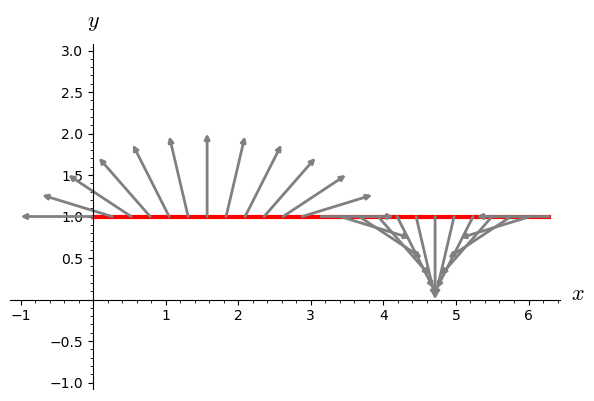Example 21.6

Now take the same $\nabla$ on the upper halfplane but consider the vertical curve $\gamma:\ x=0,\ y=t+1, \ t\in [0,1]$ and the vector field $Y=(t+1)\frac{\partial}{\partial x}.$

First let us check that $Y$ is parallel along $\gamma$.

System (21.9c) takes the form

\begin{equation} \begin{matrix} \frac{dY^1}{dt}-\frac{1}{y}Y^1=0,\\ \frac{dY^2}{dt}-\frac{1}{y}Y^2=0. \end{matrix} \tag{21.9e} \end{equation}
In :
from sympy import *          # import SymPy
init_printing()              # SymPy's latex output
t=var('t')                   # symbolic variable t
c1=lambda t:0                # first component of the  curve
c2=lambda t:1+t              # second component of the  curve
Y1=lambda t:1+t              # first component of Y
Y2=lambda t:0                # second component of Y
Y1(t).diff(t)-1/c2(t)*Y1(t)  # LHS of the first equation (21.9e):

Out:
$\displaystyle 0$
In :
Y2(t).diff(t)-1/c2(t)*Y2(t)  # LHS of the second equation (21.9e):

Out:
$\displaystyle 0$

Now solve the system (21.9e) (we have restarted the Kernel).

In :
t = symbols('t')             # symbolic variable t
Y1 = Function('Y1')          # first component of Y
Y2 = Function('Y2')          # second component of Y
ics = {Y1(0): 1,Y2(0):0}     # initial conditions
# solve system (21.9e)
# y is replaced by c2(t)=t+1
sol = dsolve([Y1(t).diff(t)-1/(t+1)*Y1(t),Y2(t).diff(t)-1/(t+1)*Y2(t)],
ics=ics)
sol                          # show solution

Out:
$\displaystyle \left[ \operatorname{Y_{1}}{\left(t \right)} = t + 1, \ \operatorname{Y_{2}}{\left(t \right)} = 0\right]$

Example 21.7

Show graphically the vector field $Y$ from the previous example along the curve $x=0,\ y=t+1.$

In :
reset()
%display latex
M = Manifold(2, 'M', start_index=1)        # manifold M, y>0
c_xy.<x,y> = M.chart()                     # chart on M
t = var('t')                               # symbolic variable t
S = M.curve([ 0,t+1], t)                   # curve S
Y = M.vector_field([t+1,0])                # vector field Y
YS = Y.along(S)                            # vector field Y along S
graph = S.plot(prange=(0, 1),thickness=3)  # plot S
graph += YS.plot(c_xy, ranges={t: (0,1)},  # plot YS
arrowsize=1.5,number_values=16,color='grey')
show(graph, ymax=2.,ymin=1,xmax=4)         # show plot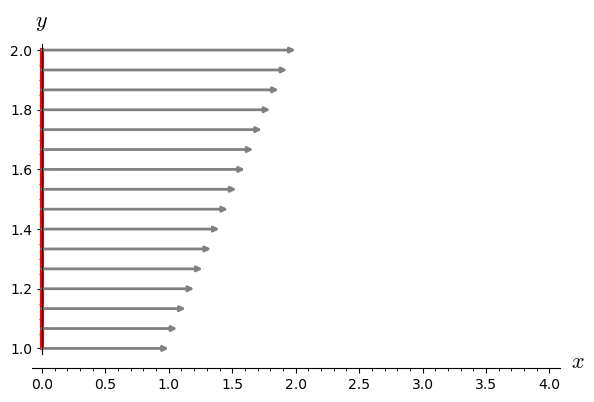Example 21.8

Consider the plane $R^2$ with Christoffel symbols $$Γ^1_{11}= Γ^1_{22}=\frac{4u}{1+u^2+4v^2},\\ Γ^2_{11}= Γ^2_{22}=\frac{4v}{1+u^2+4v^2},$$ and the remaining symbols equal to 0.

In :
reset()
%display latex
N=Manifold(2,name='R2',start_index=1)   # manifold M, dim=2
c_uv.<u,v>=N.chart()                    # coordinates u,v
nab=N.affine_connection('nab')          # connection on M
nab[:]=[[[4*u/(4*u^2 + 4*v^2 + 1), 0],  # Christoffel symbols
[0, 4*u/(4*u^2 + 4*v^2 + 1)]],
[[4*v/(4*u^2 + 4*v^2 + 1), 0],
[0, 4*v/(4*u^2 + 4*v^2 + 1)]]]
nab.display(coordinate_labels=False,only_nonzero=False)

Out:
$\displaystyle \begin{array}{lcl} \Gamma_{\phantom{\, 1}\,1\,1}^{\,1\phantom{\, 1}\phantom{\, 1}} & = & \frac{4 \, u}{4 \, u^{2} + 4 \, v^{2} + 1} \\ \Gamma_{\phantom{\, 1}\,1\,2}^{\,1\phantom{\, 1}\phantom{\, 2}} & = & 0 \\ \Gamma_{\phantom{\, 1}\,2\,1}^{\,1\phantom{\, 2}\phantom{\, 1}} & = & 0 \\ \Gamma_{\phantom{\, 1}\,2\,2}^{\,1\phantom{\, 2}\phantom{\, 2}} & = & \frac{4 \, u}{4 \, u^{2} + 4 \, v^{2} + 1} \\ \Gamma_{\phantom{\, 2}\,1\,1}^{\,2\phantom{\, 1}\phantom{\, 1}} & = & \frac{4 \, v}{4 \, u^{2} + 4 \, v^{2} + 1} \\ \Gamma_{\phantom{\, 2}\,1\,2}^{\,2\phantom{\, 1}\phantom{\, 2}} & = & 0 \\ \Gamma_{\phantom{\, 2}\,2\,1}^{\,2\phantom{\, 2}\phantom{\, 1}} & = & 0 \\ \Gamma_{\phantom{\, 2}\,2\,2}^{\,2\phantom{\, 2}\phantom{\, 2}} & = & \frac{4 \, v}{4 \, u^{2} + 4 \, v^{2} + 1} \end{array}$

Assume that the curve $\gamma$ is defined by $\ x=t,\ y=t,\ t\in (0,1)$.

System (21.9b) takes the form

$$\frac{dY^1}{dt}+\frac{4t}{1+8t^2}(Y^1+Y^2)=0,\\ \frac{dY^2}{dt}+\frac{4t}{1+8t^2}(Y^1+Y^2)=0.$$

and can be solved with Sympy (with initial conditions $Y^1(0)=1,\ Y^2(0)=0$).

In :
from sympy import *
init_printing()
%display plain
t = symbols('t')                               # symbolic variable t
w=4*t/(1+8*t**2)                               # ODE system coefficient
Y1 = Function('Y1')                            # first component of Y
Y2 = Function('Y2')                            # second component of Y
ics = {Y1(0):1,Y2(0):0}                        # initial conditions
sol = dsolve([Y1(t).diff(t) + w*(Y1(t)+Y2(t)), # solve ODE system
Y2(t).diff(t) + w*(Y1(t)+Y2(t))],ics=ics)
list(map(simplify,sol))                        # simplify components

Out:
$\displaystyle \left[ \operatorname{Y_{1}}{\left(t \right)} = \frac{1}{2} + \frac{1}{2 \sqrt{8 t^{2} + 1}}, \ \operatorname{Y_{2}}{\left(t \right)} = - \frac{1}{2} + \frac{1}{2 \sqrt{8 t^{2} + 1}}\right]$

Example 21.9

Using the data from the previous example show the parallel transport of the tangent vector at $(0,0)$ with components $(1,0)$ along the curve $x=t, \ y=t, \ t\in [0,1].$

In :
reset()
%display latex
M = Manifold(2, 'M', start_index=1)        # manifold M
c_xy.<x,y> = M.chart()                     # chart on M
t = var('t')                               # symbolic variable t
S = M.curve([ t,t], t)                     # curve S
# vector field Y:
Y = M.vector_field([0.5*(1+1/sqrt(1+8*t**2)),0.5*(-1+1/sqrt(1+8*t**2))])

YS = Y.along(S)                            # vector field Y along S
graph = S.plot(prange=(0, 1),thickness=2)  # plot S
graph += YS.plot(c_xy, ranges={t: (0,1)},  # plot YS
arrowsize=1.5,number_values=11,color='grey')
show(graph, ymax=1.1,ymin=0,xmax=2,figsize=[4,3])   # show plot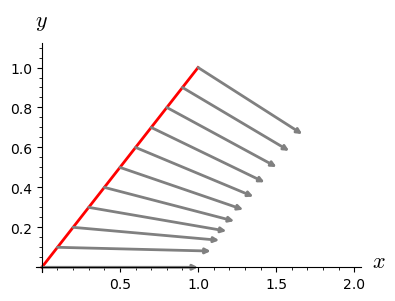Remark. In many examples, the exact solutions to ODE systems are not accessible.

Fortunately, the same figure as in previous example can be obtained, solving the system numerically.

Example 21.10

Use numerical tools to obtain the figure from the previous example.

First we check that the connection coefficients from the previous example correspond to an elliptic paraboloid.

In :
%display plain
u, v = var('u, v', domain='real')
eparaboloid = ParametrizedSurface3D([u, v, u^2+v^2], [u, v],
'elliptic paraboloid')
eparaboloid.connection_coefficients()

Out:
{(1, 1, 1): 4*u/(4*u^2 + 4*v^2 + 1),
(1, 1, 2): 4*v/(4*u^2 + 4*v^2 + 1),
(1, 2, 1): 0,
(1, 2, 2): 0,
(2, 1, 1): 0,
(2, 1, 2): 0,
(2, 2, 1): 4*u/(4*u^2 + 4*v^2 + 1),
(2, 2, 2): 4*v/(4*u^2 + 4*v^2 + 1)}

Now we can use the method

eparaboloid.parallel_translation_numerical(curve, t, v0, tinterval).

In the above command

curve =[x(t),y(t)] can be replaced for example by [s,s],

t by s,

v0 by [1.0,1.0],

and tinterval =[t_0,t_1,number_of_subintervals] by [0.0, 1.0, 9].

Use the command eparaboloid.parallel_translation_numerical? to obtain more information.

In :
s = var('s')                           # symbolic variable
vector_field = eparaboloid.parallel_translation_numerical([s,s],s,
[1.0,0.0],[0.0, 1.0, 9])   # numerical parallel translations
times, components = zip(*vector_field) # list of t_i and Y_i
vec=[vector(v) for v in components]    # vectors corresponding to Y_i
strt=[vector([t,t]) for t in times]    # vector of points on the curve
p=plot(s,(s,0,1),thickness=2,color='red')     # plot the curve in red
pl=sum([plot(vec[k],start=strt[k],arrowsize=1.5, color='grey')
for k in range(10)])           # plot all  vectors Y_i
(p+pl).show(xmax=2,ymin =-0.2,figsize=[4,3])  # combine plots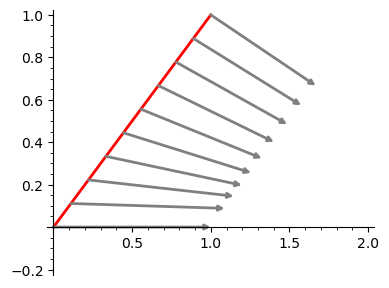Let us note that the values of the compositions $\Gamma_{ij}^k\circ\gamma$ in the above calculations are cached, and can be used for example in symbolic computations:

In :
# after above calculations the same command gives different result
eparaboloid.connection_coefficients()

Out:
{(1, 1, 1): 4*s/(8*s^2 + 1),
(1, 1, 2): 4*s/(8*s^2 + 1),
(1, 2, 1): 0,
(1, 2, 2): 0,
(2, 1, 1): 0,
(2, 1, 2): 0,
(2, 2, 1): 4*s/(8*s^2 + 1),
(2, 2, 2): 4*s/(8*s^2 + 1)}

Remark. In previous examples we have used linear functions to define $\gamma$. If the numerical approach is used we can try more complicated curves.

Example 21.11

Let us investigate the parallel transport from the previous example but along the curve $x=t,\ y=t^2, \ t\in [0,1]$.

In :
u, v = var('u, v', domain='real')        # symbolic variables for surf. param.
eparaboloid = ParametrizedSurface3D([u, v, u^2+v^2], [u, v],
'elliptic paraboloid')   #  elliptic paraboloid - parametric def.
s = var('s')                             # parameter of curve
vector_field = eparaboloid.parallel_translation_numerical([s,s^2],s,
[1.0,0.0],[0.0, 1.0, 9])         # compute parallel translations Y_i of Y_0
# for i=1,...,9
times, components = zip(*vector_field)   #  list of t_i and Y_i, i=0,...,9

vec=[vector(v) for v in components]      # vectors corresponding to lists  Y_i
strt=[vector([t,t^2]) for t in times]    # vector of points on the curve
p=plot(s^2,(s,0,1),thickness=2,color='red')       # plot curve
pl=sum([plot(vec[k],start=strt[k],arrowsize=1.5,color='grey')
for k in range(10)])             # plot all vectors Y_i
(p+pl).show(xmax=2,ymin =-0.2,figsize=[4,3])      # combine plots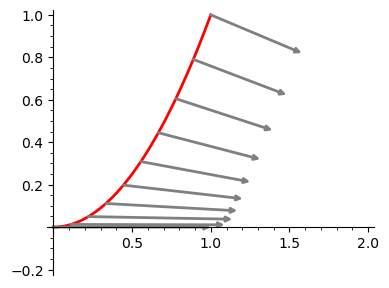Remark. This time the symbols $\Gamma_{ij}^k\circ\gamma$ are more complicated:

In :
%display plain
eparaboloid.connection_coefficients()

Out:
{(1, 1, 1): 4*s/(4*s^4 + 4*s^2 + 1),
(1, 1, 2): 4*s^2/(4*s^4 + 4*s^2 + 1),
(1, 2, 1): 0,
(1, 2, 2): 0,
(2, 1, 1): 0,
(2, 1, 2): 0,
(2, 2, 1): 4*s/(4*s^4 + 4*s^2 + 1),
(2, 2, 2): 4*s^2/(4*s^4 + 4*s^2 + 1)}

Example 21.12

Show how the equations (21.9b) of the parallel vector field along a curve can be obtained in SageMath Manifolds for a concrete parametric surface in and concrete curve.

If the equations (21.9b) of the parallel transport system are written in the form

$$\frac{dY^1}{dt}=F^1(Y^1,Y^2),\ \ \frac{dY^1}{dt}=F^1(Y^1,Y^2),$$

then the right hand sides can be obtained as follows (we use the symmetry of connection coefficients for parametrized surfaces in $R^3$).

In :
u, v = var('u, v', domain='real')     # parameters of surface
epar = ParametrizedSurface3D([u, v, u^2+v^2], [u, v],
'elliptic paraboloid')    # definition of surface

t=var('t')                            # parameter of curve
curve=[t,t^2]                         # definition of curve
surf=epar                             # renaming of surface
u1 = surf.variables                # extract the parameters
u2 = surf.variables                # from the surf object

du1 = diff(curve, t)               # derivatives of
du2 = diff(curve, t)               # curve components

C = surf.connection_coefficients()    # connection coefficients

for coef in C:                        # compositions of connection coeff
C[coef] = C[coef].subs({u1: curve, u2: curve})
# with curve components

Y1,Y2=var('Y1 Y2')                    # symbolic variables for Y components
# RHS for the first equation:
F1 = - C[(1,1,1)]*Y1*du1 - C[(1,2,1)]*(du1*Y2 + du2*Y1) - \
C[(2,2,1)]*du2*Y2
# RHS for the second equation
F2 = - C[(1,1,2)]*Y1*du1 - C[(1,2,2)]*(du1*Y2 + du2*Y1) - \
C[(2,2,2)]*du2*Y2


Thus the right hand sides of equations for parallel transport on the surface $\ \ z=u^2+v^2\ \$ along the curve $\ u=t,\ v=t^2,\$ are:

In :
%display latex
F1

Out:
$\displaystyle -\frac{8 \, Y_{2} t^{2}}{4 \, t^{4} + 4 \, t^{2} + 1} - \frac{4 \, Y_{1} t}{4 \, t^{4} + 4 \, t^{2} + 1}$
In :
F2

Out:
$\displaystyle -\frac{8 \, Y_{2} t^{3}}{4 \, t^{4} + 4 \, t^{2} + 1} - \frac{4 \, Y_{1} t^{2}}{4 \, t^{4} + 4 \, t^{2} + 1}$

Example 21.13

Make a three-dimensional illustration for parallel transport on the surface $\ \ z=u^2+v^2\ \$ along the curves $\ u=t,\ v=t,\$ and $\ u=t,\ v=t^2.\$

Since the connection from the last example corresponds to the parametrized surface $z=u^2+v^2$ we are able to make a 3d illustration of the parallel transport.

First the case of the curve $x=t, y=t$:

In :
u, v = var('u, v', domain='real')         # parameters of surface
epar = ParametrizedSurface3D([u, v, u^2+v^2], [u, v],
'elliptic paraboloid')            # definition of surface
s = var('s')                              # parameter of curve
# numerical parallel transport:
vector_field = epar.parallel_translation_numerical([s,s],s,
[1.0, 0.0], [0.0, 1.0, 9])            # compute parallel transport Y_i
# of Y_0 for i=1,..,9
times, comp = zip(*vector_field)          # list of s_i and Y_i, i=0,...,9

# plot obtained vectors:
pl=sum([plot(vector(epar.tangent_vector((times[k],times[k]),
(comp[k], comp[k]))),
start=vector((times[k],times[k],times[k]^2+times[k]^2)),color='black',thickness=2)
for k in range(8)])
# plot the curve:
p=parametric_plot3d((s,s,s^2+s^2),(s,0,0.88),thickness=4,color='red')
var('r phi')                              # polar surface parameters
# plot surface:
p1=parametric_plot3d((r*cos(phi),r*sin(phi),r^2),(r,0,1.45),(phi,0,2*pi),
opacity=0.8, color='lightgrey')
# combine plots:
(pl+p+p1).rotateZ(-1.85*pi).rotateY(0.5).rotateX(-0.65).show(frame=False)
# enlarge  to obtain a better view


and next the case of the curve $x=t, \ y=t^2:$

In :
u, v = var('u, v', domain='real')        # parameters of surface
epar = ParametrizedSurface3D([u, v, u^2+v^2], [u, v],
'elliptic paraboloid')               # definition of surface
s = var('s')                             # parameter of curve
# numerical parallel transport:
vector_field = epar.parallel_translation_numerical([s,s^2],s,
[1.0, 0.0], [0.0, 1.0, 9])  # compute parallel translations Y_i
# of Y_0 for i=1,..,9
times, comp = zip(*vector_field)         # list of s_i and Y_i, i=0,...,9

# plot obtained vectors:
pl=sum([plot(vector(epar.tangent_vector((times[k],times[k]),
(comp[k], comp[k]))),
start=vector((times[k],times[k]^2,times[k]^2+times[k]^4)),color='black',thickness=2)
for k in range(8)])
# plot the curve:
p=parametric_plot3d((s,s^2,s^2+s^4),(s,0,0.88),thickness=4,color='red')
var('r phi')                             # polar surface parameters
# plot surface:
p1=parametric_plot3d((r*cos(phi),r*sin(phi),r^2),(r,0,1.45),(phi,0,2*pi),
opacity=0.8,color='lightgrey')
# combine plots
(pl+p+p1).rotateZ(-1.95*pi).rotateY(0.5).rotateX(-0.65).show(frame=False)
# enlarge  to obtain a better view


### Geodesics¶

Recall that in (7.6) we have defined the tangent vector to a curve $\gamma$ as

$$\gamma'_{t_0}(f) =\frac{d}{dt}(\gamma^*f)\Big|_{t_0}= \frac{d}{dt}(f\circ\gamma)\Big|_{t_0},\quad f \in C^\infty(M).$$

In a manifold $M$ with a connection $\nabla,\$ geodesics can be defined as follows.

The smooth curve $\gamma: I\to M$ is geodesic if \begin{equation} \nabla_{\gamma'}\gamma'=0. \tag{21.10} \end{equation}

To obtain the equation in local coordinates we have to replace in (21.9) the components of the vector field $\ Y^k\circ\gamma\ \$ by $\ \frac{d (x^k\circ\gamma)}{dt}$ :

\begin{equation} \frac{d^2(x^k\circ\gamma)}{dt^2}+(\Gamma^k_{ji}\circ\gamma)\frac{d(x^i\circ\gamma)}{dt}\frac{d(x^j\circ\gamma)}{dt}=0. \tag{21.11} \end{equation}

Usually we shall use the shorthand

\begin{equation} \frac{d^2x^k}{dt^2}+\Gamma^k_{ji}\frac{dx^i}{dt}\frac{dx^j}{dt}=0. \tag{21.12} \end{equation}

In the case $n=2$, the system takes the form

$$\frac{d^2x^1}{dt^2}+\Gamma^1_{11}\frac{dx^1}{dt}\frac{dx^1}{dt} +\Gamma^1_{12}\frac{dx^1}{dt}\frac{dx^2}{dt} +\Gamma^1_{21}\frac{dx^2}{dt}\frac{dx^1}{dt} +\Gamma^1_{22}\frac{dx^2}{dt}\frac{dx^2}{dt} =0,\\ \frac{d^2x^2}{dt^2}+\Gamma^2_{11}\frac{dx^1}{dt}\frac{dx^1}{dt} +\Gamma^2_{12}\frac{dx^1}{dt}\frac{dx^2}{dt} +\Gamma^2_{21}\frac{dx^2}{dt}\frac{dx^1}{dt} +\Gamma^2_{22}\frac{dx^2}{dt}\frac{dx^2}{dt} =0.$$

For the standard connection of $R^n$, defined by $\Gamma_{ij}^k =$ 0 in the basis induced by the natural coordinates of $R^n$, the geodesic equations (21.11) are of the form $\frac{d^2 x^k }{dt^2} = 0$, which means that the geodesics are straight lines.

Example 21.14

Consider again the plane $R^2$ with Christoffel symbols $$Γ^1_{11}= Γ^1_{22}=\frac{4u}{1+u^2+4v^2},\\ Γ^2_{11}= Γ^2_{22}=\frac{4v}{1+u^2+4v^2},$$ and the remaining symbols equal to 0.

The system (21.12) has the form $$\frac{d^2x^1}{dt^2}+\frac{4x^1}{1+4(x^1)^2+4(x^2)^2} \Big(\big(\frac{dx^1}{dt}\big)^2+ \big(\frac{dx^2}{dt}\big)^2\Big)=0,\\ \frac{d^2x^2}{dt^2}+\frac{4x^2}{1+4(x^1)^2+4(x^2)^2} \Big(\big(\frac{dx^1}{dt}\big)^2+ \big(\frac{dx^2}{dt}\big)^2\Big)=0.$$

The exact solutions for such systems are not easy to obtain, but fortunately we can use SageMath

geodesics_numerical(p0, v0, tinterval) method,

where p0 is the list of coordinates of the initial point, v0 is the list of components of the initial tangent vector and tinterval is of the form $[t_0,t_{end},numb]$, with $numb$ denoting the number of subintervals in the interval $[t_0,t_{end}]$.

The output is a list of expressions of the form:

[t, [u1(t), u2(t)], [v1(t), v2(t)], [x1(t), x2(t), x3(t)]],

where t denote the ends of subintervals of $[t_0,t_{end}],$

[u1(t), u2(t)] are the intrinsic coordinates of the geodesic points,

[v1(t), v2(t)] are the intrinsic coordinates of the tangent vectors to the geodesic,

[x1(t), x2(t), x3(t)] are the coordinates of the geodesic points in the three-dimensional space.

Use

In :
# epar.geodesics_numerical?


In the following code we compute numerically the geodesic with initial tangent vector at $(u1,u2)=(-1,0)$, with components $(v1,v2)=(0,-1).\$ In the computations, the time interval $[0,120]$ is divided into 200 subintervals:

In :
u, v = var('u, v', domain='real')  # parameters of surface
epar = ParametrizedSurface3D([u, v, u^2+v^2], [u, v],
'elliptic paraboloid')     # definition of surface
s = var('s')                       # parameter of curve
# numerically solve the
# equations of geodesics:
geodesic = epar.geodesics_numerical([-1,0.],[0.0,-1],
[0.0, 120, 200])
# 'geodesic' contains times t_i, points p_i, tang.vects v_i
#  and coordinates (x_i,y_i,z_i) of points on geodesic
times,points,tang,ext = zip(*geodesic)
p0=line3d(ext,thickness=4,color='red')  # plot the geodesic
r,phi=var('r phi')                 # polar parameters of surface
p1=parametric_plot3d((r*cos(phi),r*sin(phi),r^2),(r,0,11),
(phi,0,2*pi),opacity=0.5,color='lightgrey')   # plot surface
(p0+p1).rotateZ(0.4*pi).show(frame=False,aspect_ratio=[1,1,0.2])
# enlarge and rotate for better view


Example 21.15

Show three examples of geodesics on the sphere in $R^3$.

Define the sphere as the parametric surface

$$(x,y,z)=(\cos(u)\cos(v), \cos(v)\sin(u), \sin(v)),\\ u\in (0, 2\pi),\ \ v\in (-\pi/2, \pi/2).$$
In :
S = surfaces.Sphere()                 # use surfaces.Sphere module
S.equation                            # show equations of unit sphere

Out:
$\displaystyle \left(\cos\left(u\right) \cos\left(v\right), \cos\left(v\right) \sin\left(u\right), \sin\left(v\right)\right)$
In :
S.variables_range                     # show ranges of variables

Out:
$\displaystyle \left(\left(0, 2 \, \pi\right), \left(-\frac{1}{2} \, \pi, \frac{1}{2} \, \pi\right)\right)$

and compute numerically sets of 101 points on three geodesics, each starting at point $(u,v)=(0,0)$ and having initial tangent vectors with components $\ (1,0),\ (\cos(\pi/3),\sin(\pi/3),\ (\cos(-\pi/3),\sin(-\pi/3),\$ respectively:

In :
# 101 points of the geodesic with initial tangent vector
# at point (u,v)=(0,0) with components (1,0)
g1 = [c[-1] for c in S.geodesics_numerical(
(0,0),(1,0),(0,2*pi,100))]

# 101 points of the geodesic with initial tangent vector
# at point (u,v)=(0,0) with components (cos(pi/3),sin(pi/3))
g2 = [c[-1] for c in S.geodesics_numerical(
(0,0),(cos(pi/3),sin(pi/3)),(0,2*pi,100))]

# 101 points of the geodesic with initial tangent vector
# at point (u,v)=(0,0) with components (cos(2pi/3),sin(2pi/3))
g3 = [c[-1] for c in S.geodesics_numerical(
(0,0),(cos(-pi/3),sin(-pi/3)),(0,2*pi,100))]

p0=S.plot(opacity=0.5,color='lightgrey') # plot sphere
p1=line3d(g1,thickness=4,color='red')    # first geodesic
p2=line3d(g2,thickness=4,color='red')    # second geodesic
p3=line3d(g3,thickness=4,color='red')    # third geodesic

(p0+p1+p2+p3).rotateZ(-pi/4).show(frame=False) # combine plots